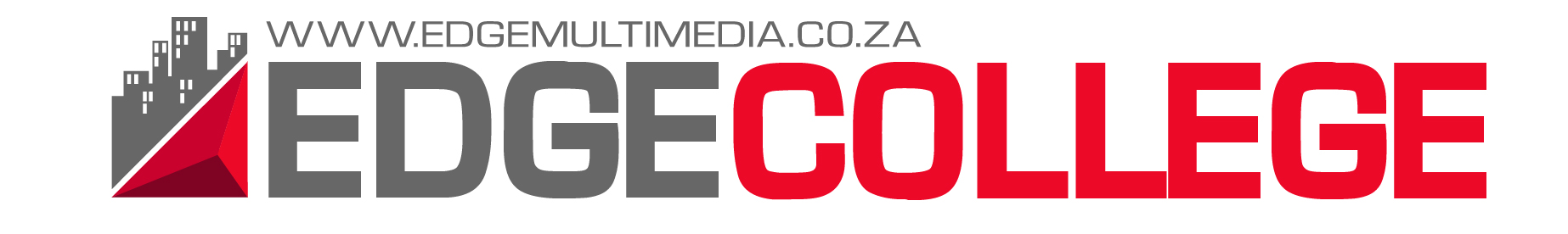Course Outline:

Course Description:

Interferometry is a fundamental technique used in radio astronomy but is also a fairly unique technique which can lead to difficulties the first time it is introduced. Though, it is a subject which is based in fundamental concepts of mathematics. Thus, we should not be scared of it. The focus of this course is to start from a point of interest in understanding interferometry and get us to a place where we are comfortable enough in the fundamentals that we can move onto the unique complexities that arise in the use of these arrays to make scientific observations.

We will start by motivating the use of interferometric arrays for doing radio science. From there we will cover the mathematical basis for the technique. Using this basis we will cover the process of imaging and deconvolution. With the theoretical groundwork covered, we will then discuss the practical topics of using interferometric arrays including observing systems, calibration, and standard reduction software. The course will be taught using practical, interactive lectures and requires knowledge of basic python and linux. Marks will be based on a practical calibration and imaging project using KAT-7 data which will make up the final portion of the course and small assignments.

Syllabus:

1. Motivating interferometry for radio astronomy science
2. Mathematical ground work: Fourier Transforms and filtering, convolution and correlation, Jones and Mueller Calculus, Least-Squares Fitting
3. Positional Astronomy: the Celestial Sphere, Sidereal Time, and coordinate systems
4. Visibility Space: the visibility equation and the van Cittert-Zernike Theorem, uv-space, rotational synthesis
5. Imaging: spatial filters, sampling and weight functions, deconvolution, source finding
6. Observing Systems: the Measurement Equation, system electronics, antenna beams and feeds, polarization, propagation effects
7. Calibration: classic calibration, self-calibration methods
8. Practical: reduction of a interferometric measurement data set by applying flagging, calibration, imaging, and deconvolution techniques to create a final image

We will use https://github.com/griffinfoster/fundamentals_of_interferometry as the basis of the course.

X